## NCERT Solutions for Class 7 Maths Term 1 and Term 2 [Updated 2021-22]

Maths is one of the most important subjects for high school students as it covers all fundamentals topics in a comprehensive manner. So, students are advised to download NCERT Solutions for Class 7 Maths PDFs Chapter-wise from the available links and kickstart the preparation.

Practice each and every concept of the 7th class math chapters and solve the problems effectively in the final CBSE 7th Maths Board Exam. Our subject experts designed these chapterwise PDF formatted NCERT 7th Maths Solutions in a simple language based on the NCERT Textbooks and the latest CBSE Guidelines.

## CBSE NCERT Solutions Class 7 Maths Chapter-wise & Exercise-wise Free PDF Download

Students who seem emphasized seeking step-by-step and detailed NCERT Maths Textbook Solutions for Class 7 can get them at NCERTBooks.Guru in a very explanative manner. So, download the NCERT Solutions for Class 7th Maths by going through the below exercise-wise chapters links and start preparing all the concepts of mathematics efficiently.

All these exercises questions and answers are formed by our subject teachers to help students during exam preparation and achieve their academic goals with good scores. Simply click on the respective chapter links of Maths NCERT 7th Class Solutions PDFand prepare thoroughly for your examinations.

### Chapter 1 Integers

One of the main concepts in Mathematics is Integers. Studying more about the major topic will guide solve the related problems so easily at any time and also help in facing higher classes concepts. In this chapter, you are going to learn the exercise-wise concepts like properties and operations of integers, the addition and the subtraction of the integers, properties of addition and subtraction of integers, multiplication & division of integers, properties of multiplication and division of integers.

### Chapter 2 Fractions and Decimals

NCERT Book Solutions for Class 7 Maths Chapter 2 Fractions and Decimals start explaining the concepts of the operations of multiplication and division on fractions as well as on decimals. Moreover, you can continue your practice with the topics covered in these exercises like multiplication of a fraction by a whole number, multiplication of a fraction by a fraction, division of the whole number by a fraction, division of a fraction by a whole number, multiplication of decimal by 10,100, 100, etc.

### Chapter 3 Data Handling

Students who feel risky to learn how to do data handling can go with these exercises and come across with some more kinds of data and graphs. Moreover, you will also understand how to organize experiences and draw inferences from them.

### Chapter 4 Simple Equations

NCERT Textbook solutions of 7th maths chapter 4 simple equations covers the concepts like setting up an equation, solving an equation, transposing a number, applications of simple equations to practical situations. Below are exercise solutions links of the ch 4 simple equations NCERT Solutions Maths.

### Chapter 5 Lines and Angles

This chapter explains various types of angles at length. Concepts like elated angles, complementary angles, supplementary angles, adjacent angles, linear pair, vertically opposite angles, pairs of lines, intersecting lines, transversals, the angle made by a transversal, transversal of parallel lines, and checking of parallel lines are also covered in the chapter 5 Lines and Angles Class 7 NCERT Textbook Maths Solutions.

### Chapter 6 The Triangle and its Properties

Class 7 Maths NCERT solutions chapter 6 the triangle and its properties deal with the topics like Drawing the median and altitude of triangles, determining the exterior angles, and finding the value of unknown angles using the angle sum property, and many more. It provides students a detailed knowledge of the elements of a triangle. Furthermore, this chapter also covers an illustration of the isosceles and equilateral triangles.

### Chapter 7 Congruence of Triangles

Here, you have seen the provided chapter 7 exercises solutions links where students introduced a term known as “congruence”. Students will study the major topics like Congruence of plane figures, congruence among line segments, congruence of angles, congruence of triangles, criteria for congruence of triangles, congruence among right-angled triangles in this chapter.

### Chapter 8 Comparing Quantities

Class 7 Maths Chapter 8 describes the importance of comparing two quantities in our everyday life. They may be heights, weights, salaries, marks, etc. From this chapter, students will understand the method of using ratios for the comparison of the given quantities.

Two ratios can be compared by converting them to like fractions. We say the two given ratios are equivalent when the two given fractions are equal. Fun with estimation, interpreting percentages, converting percentages to how many, ratios to percents, increase or decrease as percent, profit or loss as a percentage, charge given on borrowed money or simple interest are few other interesting topics covered in this chapter.

### Chapter 9 Rational Numbers

The Rational Numbers Chapter deals with the topics like positive and negative rational numbers, rational numbers on a number line, rational numbers in standard form, comparison of rational numbers, rational numbers between two rational numbers, operations on rational numbers. The provided 2 exercises include problems related to these concepts.

### Chapter 10 Practical Geometry

Training on how to properly use geometrical instruments to construct transversals and parallel lines, building triangles using the SSS, SAS, ASA, and RHS criteria are the concepts covered in the 5 exercises of the practical geometry chapter.

### Chapter 11 Perimeter and Area

Perimeter is the distance around a closed figure while the area is the part of a plane or region occupied by the closed figure. In this class 7 Maths NCERT Solutions Chapter 11, you will learn directly how to find the perimeter and area of different geometrical shapes. Also, it includes converting units and applying the topics discussed here to real-life problems.

### Chapter 12 Algebraic Expressions

NCERT Solutions of Class 7 Maths Chapter 12 Algebraic Expressions deals with the knowledge of some algebraic terms such as coefficients, like and unlike terms, monomials, binomials, trinomials, polynomials, addition and subtraction of algebraic expressions, finding the values of an expression, and more. All these concepts are explained in these exercise-wise solutions links. So, download and start preparing them for free & easily.

### Chapter 13 Exponents and Powers

Dealing with the exponents’ concepts is not so easy as it should be applied with various rules. Students should know that Exponents are the power of the number. So, in this chapter, you will study the multiplication, addition, division, etc. of numbers having exponents. All the concepts are covered in the below chapter 13 exercises with detailed solutions.

### Chapter 14 Symmetry

Here, the geometrical concept of symmetry is presented. Lines of symmetry for regular polygons and rotational symmetry are explained in this chapter. So, students are advised to download all the concepts by going through these three exercise-wise solutions links.

### Chapter 15 Visualising Solid Shapes

The ending part of the Class 7 Maths NCERT Solutions is the Visualising solid shapes Chapter. From here, you will study the concepts like how to classify figures in terms of dimensions, what are plane figures and what are solid shapes, what are 2D and 3D figures, top view of figures, side view, etc.

### MCQ Questions for Class 7 Maths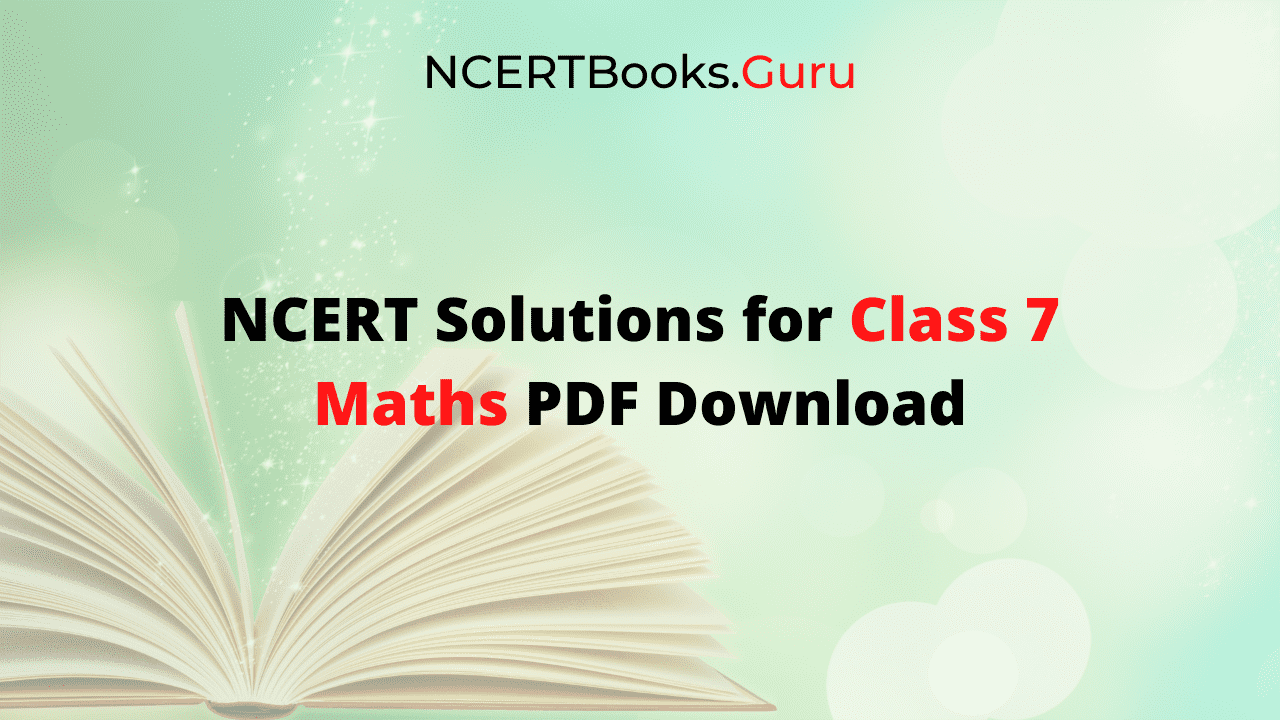### Importance of NCERT Solutions of Class 7 Maths PDF

There are countless advantages of referring to the NCERT Solutions of 7th Class Maths and they are as such:

• Understanding & solving the Maths concepts can be quite tricky but with the help of NCERT 7th maths solutions pdf, it will be easy for all students.
• All the NCERT Book Solutions for Class 7 Maths are available for free access and download offline to practice at any time and anywhere.
• By using this self-evident NCERT 7th Std Solutions, you can have a solid grip on the basic concepts of maths and get a foundation for further classes too.
• Our in-house subject matter experts have created the 7th Class NCERT Maths English and Hindi Medium as per the latest & updated 2021-22 academic year CBSE curriculum & syllabus.

### FAQs on NCERT Book Class 7 Maths Solutions Free PDF Download

1. What is the best reference material to score more marks in CBSE Class 7 Maths Exam?

NCERT Solutions for Class 7 Maths is the best reference material that covers all chapters and exercise questions and answers in a comprehensive manner. By practicing all these solutions thoroughly can help you score more in the 7th maths exams.

2. Where can I get the Solutions for 7th class maths NCERT Textbook Questions?

The detailed and step-by-step solutions for 7th class maths NCERT Textbook Questions are designed by subject experts as per the latest & updated CBSE Guidelines and you get them on our page at NCERTBooks.Guru

3. How many Chapters are there in the Class 7 Maths Textbook?

There are 15 Chapters present in the Class 7 Math Textbook.

### Conclusion

We believe the information shared about NCERT Solutions of Class 7 Maths has helped you a lot. Need any other suggestions or assistance from us please comment below so that we can get back to you soon with the proper solution. Meanwhile, visit or bookmark our site to get the latest updates on NCERT Books, Previous Question Papers, MCQ Questions, etc.

## NCERT Solutions for Class 10 Foundation of Information Technology PDF Download

NCERT Solutions for Class 10 Foundation of Information Technology Free PDF Download will be a great help for all students who are dreaming to start their career in the IT Industry. Preparaing yourself for information technology in class 10 is the best time to grasp and understand the fundamentals.

To improve your conceptual knowledge, NCERT Solutions Class 10 Foundation of Information Technology is the perfect solutions book. It provides a complete view of the prescribed syllabus. Furthermore, the knowledge of concepts becomes simple and in-depth by using the NCERT 10th Information Technology Solutions PDF Chapter-wise.

## Updated 2021-22 NCERT Solutions for Class 10 Foundation of Information Technology PDF Free Download Chapter wise

CBSE Class 10 NCERT Solutions for Foundation of Information Technology is very crucial for the CBSE students to learn the basics of IT. One of the best ways to score high in the competitive & annual exams is by solving questions covered in the NCERT Foundation of Information Technology Books.

With the NCERT Class 10th Foundation of Information Technology Solutions PDF, you can easily assess your strong and weak areas and bridge the knowledge gap with great practice. Just tap the links available here and download chapterwise NCERT 10th Std Information Technology Solutions in PDF format.Importantly, the CBSE Class 10 Foundation of Information Technology NCERT Solutions Book is the major reference material for the students planning for competitive examinations like NRRT, JEE Main/Advanced, etc. Make sure to access the chapterwise NCERT solutions of class 10 Foundation of IT subject and get a strong foundation for every chapter along with a smooth & easy knowledge of high-level concepts.

### Why is CBSE 10th Foundation of Information Technology NCERT Textbook Solutions PDF Important?

Some of the points which are helpful & important to know about why we use the NCERT Class 10 Foundation of Information Technology Solutions for preparation are as follows:

• Students who feel stress with the CBSE updated exam pattern and curriculum can deal & get out of that situation by using the 10th Class NCERT Solutions Book.
• NCERT Textbooks and Solutions books are essential for students to get proper guidance while preparation and gain more subject knowledge.
• You can acquire detailed, Step-by-Step NCERT Solutions for Class 10 Foundation of Information Technology explained by Subject Experts based on the NCERT (CBSE) Book guidelines.

### FAQs on NCERT Solutions Class 10 Information Technology Free PDF

1. How many chapters of NCERT Solutions are covered in the Class 10 Foundation of Information Technology Book Solutions?

There are 9 chapters present in the textbook of NCERT 10th foundation of information technology so you will find the chapterwise questions and answers in the NCERT Solutions of Class 10 Information Technology Book.

2. Why try NCERT Solutions Class 10 Foundation of Information Technology?

There are solid reasons to try out the NCERT Solutions Class 10 Foundation of Information Technology. As they are curated with perfect quality of solutions, errorless answers with 100% reasoning, written in a simple and easy-to-understand language, provides in-depth knowledge of every concept with exercise questions and answers, etc.

3. How to download PDF formatted NCERT Textbook solutions for 10th class foundation of information technology?

Simply click on the links available on our page and download the NCRETBooks.Guru provided free PDF formatted NCERT Textbook solutions for 10th class foundation of information technology chapterwise.

### Conclusion

We as a team hoping that the knowledge shed above concerning the NCERT Solutions Class 10 Information Technology PDF has been advantageous to you while preparation. If you have any queries, drop a comment via the comment box and we will help you out at the earliest possible. Meanwhile, visit or bookmark our site by pressing (Ctrl+D) and avail latest updates on NCERT Books, NCERT Solutions of other classes, Syllabus, Study Notes, etc.

## NCERT Solutions Class 8 English PDF Free Download | Chapterwise NCERT 8th English Honeydew & It So Happened Book Solutions PD

The guide that you are looking for is here ie., NCERT Solutions for Class 8 English (Honeydew & It So Happened) PDF in chapterwise. Download all chapter’s questions and answers from the updated 2021-22 NCERT Solutions of 8th English honeydew and it so happened books and ace up your preparation. All the Solutions available here are explained in a simple manner, according to the latest CBSE syllabus by our subject experts.

So, access the detailed solutions of the “Honeydew” and Supplementary textbook “It So Happened” chapterwise for free from the NCERT Class 8 English Textbook Solutions PDF and score well in your final exams.

## Class 8 English Honeydew & It So Happened NCERT Solutions Free PDF Download

Are you seeking homework help to finish your complex concepts tasks during learning? Then this NCERT 8th English Solution Book will help you in completing your homework within no time and make you understand the concept thoroughly. NCERT Solutions for Class 8 English Honeydew includes various units of prose and poems with exercise and activity-based questions based on the NCERT Class 8 English Textbook Syllabus.

The following are the pdf links to the unit-wise or chapter-wise NCERT 8th Class English Solutions of the main textbook Honeydew and the Supplementary textbook, It So Happened used for accessing online or download offline for further preparations.

### NCERT Solutions for Class 8 English Honeydew Chapter-wise

NCERT Solutions for Class 8 English are part of NCERT Solutions for Class 8. Here we have given CBSE English Honeydew Chapters and Poems NCERT Solutions Class 8 in PDF links. Hence, grab the opportunity of free download and start preparing for improving your knowledge and grammar skills.

### CBSE NCERT Solutions for Class 8 English It So Happened

Here we have also curated the list of chapterwise solutions for NCERT Class 8 English supplementary It So Happened Textbook. Please go with these 10 chapters of It so Happened book and try to attempt all the questions asked in the exams after preparing the solutions from here. Also, you can Download Free NCERT Textbooks PDF from our site Ncertbooks.Guru for all classes 1 to 12.

NCERT Solutions for Class 8 English Supplementary It So Happened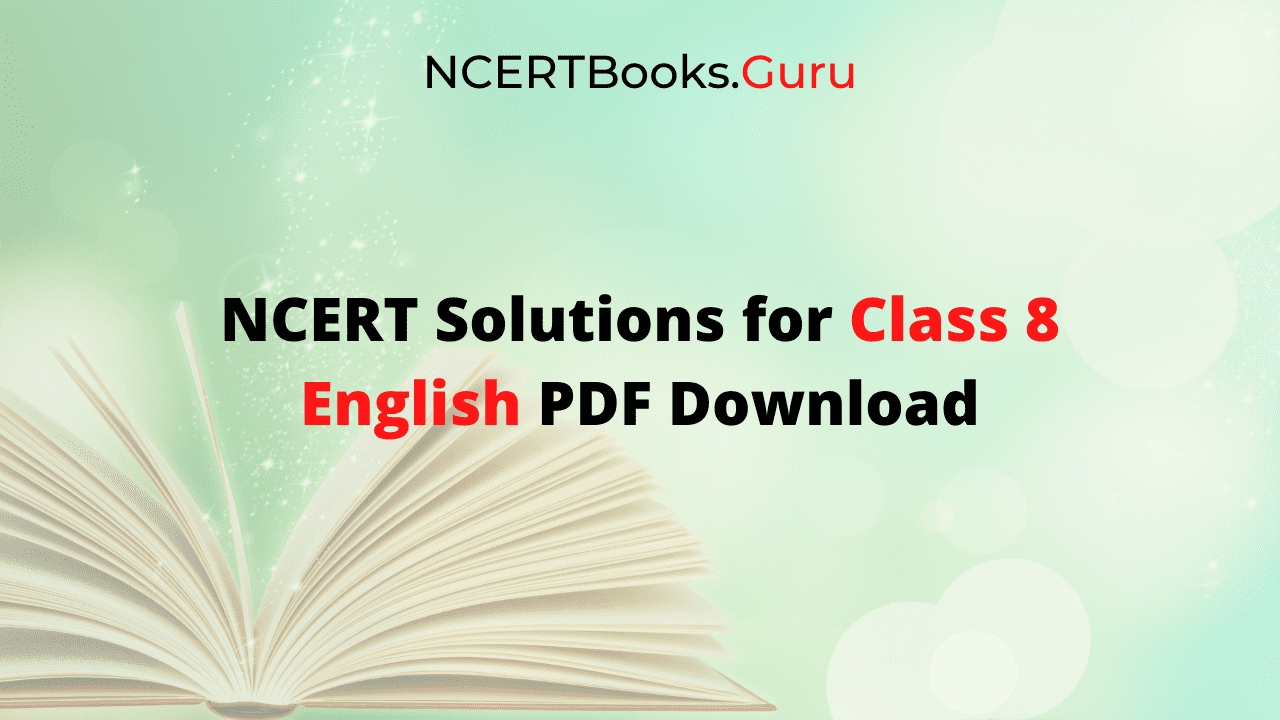### FAQs on NCERT Class 8 English Honeydew & It So Happened Solutions PDF

1. How many books are there in CBSE Class 8 English?

There are two books for 8th class English students which are prescribed by CBSE. They are:

2. Supplementary Reader – It so happened

2. Where can I download NCERT Solutions for Class 8 English Honeydew & It So Happened Books?

The NCERT Solutions for Class 8 English honeydew and it so happened books can be downloadable from our portal NCERTBooks.Guru online/offline in pdf format for free of cost. These solutions are prepared by our subject matter experts in a simple language for understanding the concepts easily to students and they are created as per the NCERT textbook for Class 8 English and the latest CBSE guidelines.

3. How many chapters are there in NCERT Class 8 English Supplementary Book It So Happened?

There are 10 chapters in NCERT Solutions of Class 8 English Supplementary Textbook, It So Happened Solutions. Unit 1- How The Camel Got His Hump, Unit 2- Children At Work, Unit 3- The Selfish Giant, Unit 4- The Treasure Within, Unit 5- Princess September, Unit 6- The Fight, Unit 7- The Open Window, Unit 8- Jalebis, Unit 9- The Comet — I, Unit 10- The Comet — II.

Kids who are excited to score well in their exams should practice consistently with the best study resources. To make the studying process easy for students, we have come up with the perfect study resources ie., Online NCERT Solutions for class 7 science PDF chapterwise.

At NCERTBooks.Guru, students will get to learn all the concepts in a detailed way with correct answers. Hence, they can secure the highest marks in the examinations. So, download the NCERT class 7 science textbook solutions in pdf format and start revising the complete syllabus with ease.

Students by referring to the NCERT 7th Science Solutions Book can self-analyze their preparation standards and results after attempting the questions in practice tests. All questions are explained in a comprehensive manner to understand by students and score maximum marks in the exams.

Also, these questions and answers are designed here as per the latest & updated CBSE Class 7 Syllabus & Exam Pattern. So, click the quick links available here and download the pdf formatted CBSE Class 7 Science NCERT Solutions.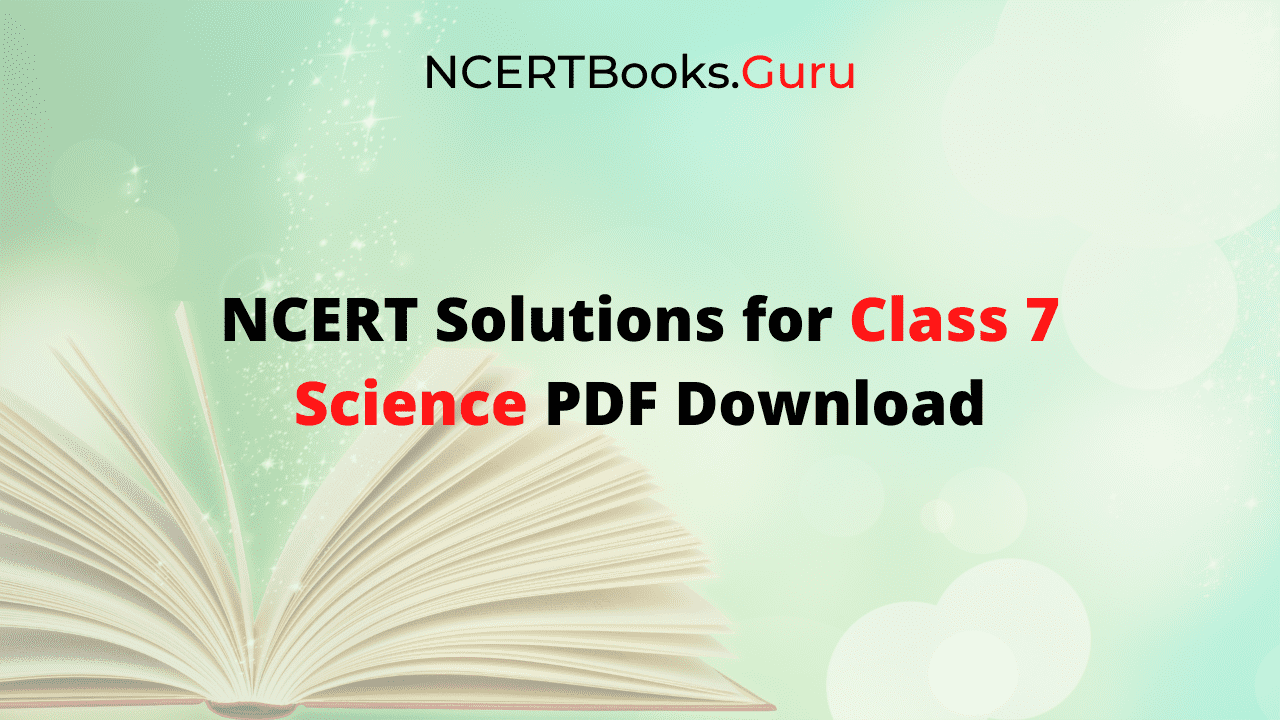• In the solution book of NCERT Class 7 Science, you will find all the answers for chapterwise questions as well as practice sets given at the end of the chapters in NCERT Textbooks.
• The questions and solutions covered in this guide will help in completing the homework and clear all your subject doubts.
• Furthermore, the answers explained in step-wise manner aid you get a better approach to frame the answers while asked in board exams.
• The language that is used while answering the questions is very interactive and easy so you all can get a stronger grip over the concepts.

### FAQs on CBSE Class 7 Science NCERT Solutions Physics PDF

1. How to score high marks in CBSE Class 7 Science Board Exams?

Ncertbooks.Guru has compiled the most comprehensive NCERT Solutions for class 7 science. It helps kids to study well and gain more subject knowledge. Having a great grip on the subject lets you score high marks in board exams.

2. Which is the best Website providing the Latest CBSE Prescribed Syllabus for Class 7 Science?

Ncertbooks.Guru is the trusted platform that offers the best solution for students like NCERT Solution for Class 7 Science as per the CBSE prescribed syllabus. So, get them now and start your effective preparation for board exams.

3. How can I download NCERT 7th Science Textbook Solutions in PDF?

By tapping the links of chapterwise NCERT 7th science book solutions available on our page, will make you download them freely in pdf format.

### Summary

We think the data bestowed here on NCERT Solutions for Class 7 Science PDF will help you to the possible extent. If you need more study resources like MCQ Questions for 7th Science with Answers, NCERT Books for Class 7, physics lecture notes, etc. comment us below and we’ll get back to you with the possible solutions asap.

Also, NCERTbooks.guru provides you the quality books that too absolutely free. So, visit the site and avail all the latest study materials and NCERT Solutions of other classes for better preparation.

## Download Free PDF Formatted NCERT Solutions of Class 6 Science Book Chapter-wise

Looking for step-by-step solutions for all the questions in the NCERT Textbooks of Class 6 Science. Here is the perfect guide to solve your worries ie., NCERT Solutions for Class 6 Science PDF. With the help of these CBSE Class 6 Science NCERT Textbook Solutions, students will learn science concepts proficiently. Moreover, you can use this ultimate study resource to revise the entire syllabus smartly before exams and attempt all the questions confidently. Get more details on the same from the further modules like pdf links, benefits, etc.

## Class 6 Social Science NCERT Textbook Solutions – Free PDF Download

From our website NCERTBooks.Guru, students can download NCERT Solutions for Class 6 Science chapters in a hassle-free way. However, you will get them free of cost and in pdf format for easy access at any platform. Instead of Science NCERT 6th Class Solutions Book, you can also access to NCERT Solutions for Class 6 Social Science, Maths, English, Hindi, etc. Just tap the links of NCERT Class 6 Science Solutions PDF and revise the complete syllabus to score more marks in your examinations.

The above pdf links of NCERT Solutions for 6th science are the reliable materials to learn and self-analyse the performance of the students. NCERT provides text book and solutions books as per the latest CBSE Syllabus & Curriculum.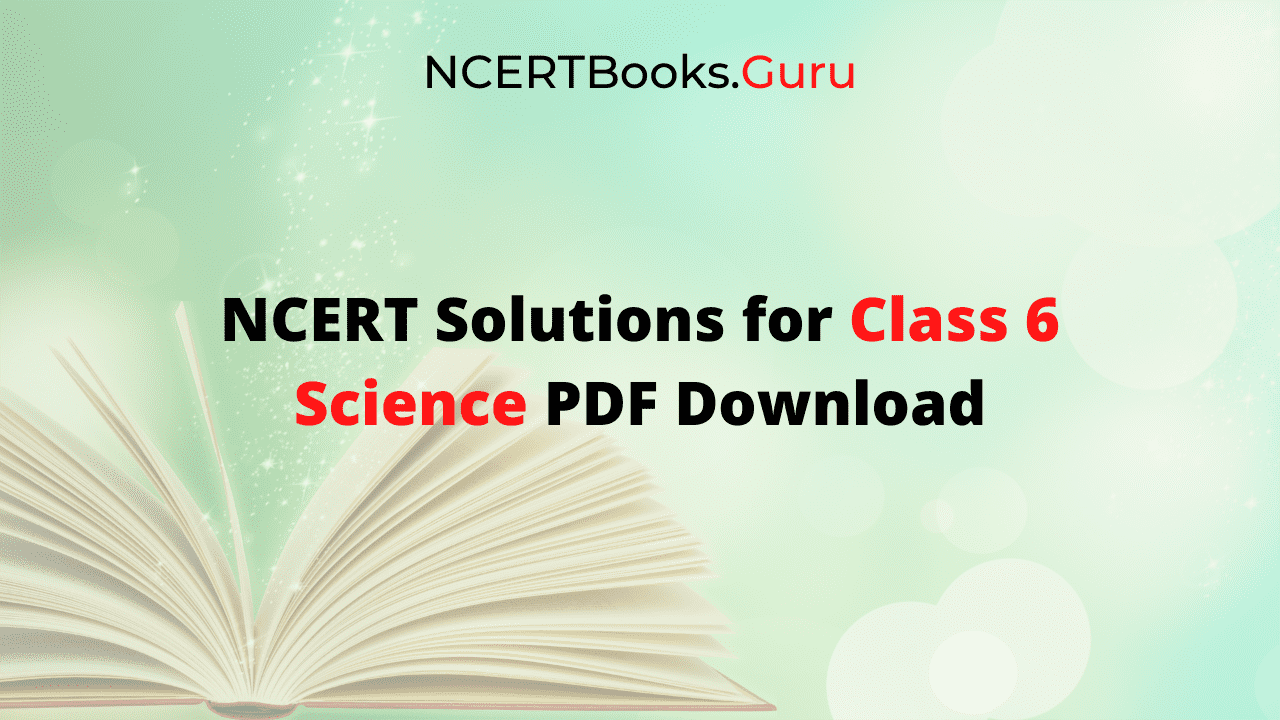So, students should likely follow the NCERT Books and NCERT Solutions for Class 6 Science PDF to practice all chapters concepts and score more marks in exams. Access the PDF format of the NCERT Solutions for Class 6 Science for free and get a good grip on all the sixteen chapters covered in the NCERT Science Textbook.

### FAQs on Class 6 NCERT Science Book Solutions PDF

1. How to study Class 6 Science Subject proficiently?

Directly refer to the NCERT Solutions provided by Ncertbooks.Guru and understand the concepts of class 6 physics & chemistry very proficiently. Also, it helps students to finish their homework or assignments at a pace.

2. How many chapters are included in NCERT 6th Science Textbook Solutions?

The chapters included in NCERT class 6 Science textbook solutions are of total sixteen. So, get deeper insights on the concepts by referring to the CBSE 6th Science NCERT Solutions PDF.

3. Will I get detailed answers to the textbook questions in PDF NCERT Class 6 Science Solutions Book?

Surely students will get detailed answers to the textbook questions in PDF of NCERT Class 6 Science Solutions Book by NCERTBooks.guru and all the answers are curated by subject experts in a simple language. So, it improves confidence among students to take up the annual exams.

### Conclusion

From this web page of NCERT Solutions for Class 6 Science PDF, you will find easy to access & downloadable pdf links of all chapters’ questions and answers. Apart from NCERT Book Solutions, other textbook solutions for all classes, NCERT Books, Notes, Question Papers are also available on NCERTBooks.Guru for free. If any of the links failed to download or show an error, feel free to drop your comments below so that will fix as soon as possible.

## NCERT Solutions for Class 6 Maths Term 1 and Term 2 [Updated 2021-22] | Chapterwise Class 6th NCERT Book Solutions for Maths

NCERT Solutions for Class 6 Maths has detailed explanations for all the questions in the Maths Textbooks prescribed by the CBSE Board. We offer Class 6th Maths NCERT Solutions for Students to resolve their doubts as well as to provide them deeper insights into the subject. All the 6th Class NCERT Book Solutions are provided in PDF Formats so that you can download them and prepare offline too in no time.

We have mentioned the Chapterwise Class 6th Maths NCERT Book Solutions for your reference. Practice the different kinds of questions in the NCERT Solutions that are likely to be asked in exams. Subject experts have designed the NCERT Books Class 6 Maths in a structured format in order to ensure proper knowledge of concepts. Have a strong foundation for further classes by practicing the 6th Std Maths NCERT Solutions PDF.

### Chapter 1 Knowing Our Numbers

In this chapter, you will learn various concepts like large numbers up to 1 crore, estimation of large numbers and roman numerals, Indian and universal arrangement of numeration. Know how to read and write and compare large numbers. All the 3 exercises present in the chapter cover these topics.

### Chapter 2 Whole Numbers

The chapter begins with the introduction of Whole Numbers, predecessor, and successor of a natural number, addition, subtraction, and representation of natural numbers on a number line. There are 3 exercises in the chapter covering these topics.

### Chapter 3 Playing with Numbers

This Chapter deals with topics like factors and multiples of a given number. Along with this concepts, you will also find topics like Perfect Number, Prime, Co-Prime Numbers, Composite Numbers, Common Factors, Common Multiples. Students of 6th Std will also become familiar with topics like finding LCM, HCF using Prime Factorization Method. All the 7 Exercises present in the Chapter include problems based on these topics.

### Chapter 4 Basic Geometrical Ideas

Students will learn about the topics line segment, type of lines, the distance between two points, concepts based on angles, polygons, regular polygons, triangles, quadrilaterals, circles. There are 6 Exercises in total in this chapter.

### Chapter 5 Understanding Elementary Shapes

This chapter tries to make students familiar with all types of shapes formed using curves or lines. In addition, you will learn how to observe edges, corners, planes, open curves, and closed curves in the surroundings. All the 9 Exercises present in the chapter covers problems on these topics so that you can better understand them.

### Chapter 6 Integers

Chapter 6 of Class 6th Maths will give you an idea of negative numbers, representation of integers on a number line, addition, and subtraction of integers using a number line, ordering of integers, etc. There are 3 exercises in this chapter to cover these topics.

### Chapter 7 Fractions

Class 6 Chapter 7th mainly covers the concepts like a number line, proper fractions, improper fractions, and mixed fractions, like fractions, the simplest form of a fraction, addition and subtraction of fractions, comparing fractions, comparing like fractions, comparing unlike fractions, etc. There are 6 exercises in this chapter and covers problems on these topics.

### Chapter 8 Decimals

The Decimals chapter deals with concepts like Tenths, Hundredths, Money, Length, Weight, Comparing and using Decimals, the Addition of numbers with Decimals, and Subtraction of Decimals. The 6 exercises present covers problems related to these topics.

### Chapter 9 Data Handling

Data Handling Chapter of Class 6th makes students familiar with concepts like data, organization of data, recording data, introduction to the idea of pictograph, how to draw a pictograph, interpretation of a pictograph, introduction to a bar graph, interpretation of a bar graph presentation and drawing a bar graph. Students will better understand the concepts of Data Handling. 4 Exercises present will help you understand these concepts in a much better way.

### Chapter 10 Mensuration

In the Mensuration Chapter, students will get to know the measurement of shapes, figures depending on area and volume.  Furthermore, you will get to know the topics like measurement of the perimeter, the perimeter of regular shapes, measurement of area, area of square and rectangle, etc. All the 3 Exercises will include problems on the listed topics.

### Chapter 11 Algebra

Through Algebra students will, l learn how to solve problems using various Alphabets. Concepts like Introduction to Algebra, matchstick patterns, the idea of a variable, examples of variables, and use of variables in common rules, using expressions practically, expressions with variables, equation, Solutions of an equation are explained in detail here. There are a total of 5 exercises in chapter 11.

### Chapter 12 Ratio and Proportion

Students will know that comparison by division is called ratio. Topics like if two ratios are equivalent or not, the idea of proportion, examples related to it, examples on unitary method, etc.  3 exercises present in the chapter will help students better understand these topics.

### Chapter 13 Symmetry

This Chapter will help you learn how to determine symmetrical items, operations based on symmetry. Principal Topics covered in this chapter are Making Symmetric Figures- Ink-blot Devils, Figures with Two Lines of Symmetry, Figures with Multiple (more than two) Lines of Symmetry, Reflection, and Symmetry. The 3 exercises better explain these concepts.

### Chapter 14 Practical Geometry

Students will learn how to make shapes and the tools used for making these shapes, etc. Concepts like the circle, construction of a circle when its radius is known, a line segment, construction of a line segment of a given length, constructing a copy of a given line segment. In addition to these you will know about perpendiculars, perpendicular to a line through a point not on it, perpendicular to a line through a point on it, the perpendicular bisector of a line segment, angles, constructing an angle of a given measure, constructing a copy of an angle of unknown measure, bisector of an angle, angles of special measures.

Students can use our MCQ Questions for Class 6 Maths to complement their preparation. Assess your preparation standards and understand where you went wrong. Bridge the knowledge gap and score well in your board exams. Attempt them on a regular basis and enhance your speed and accuracy in the final exam.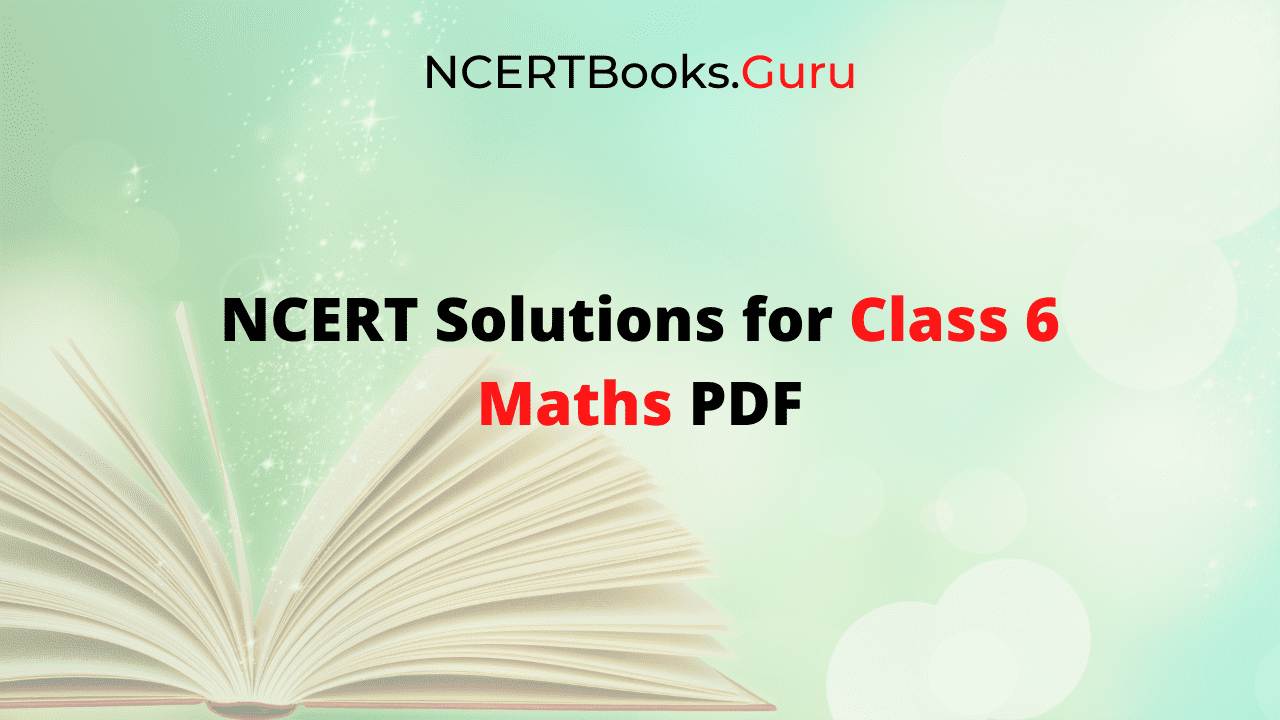Students often need help during their exam preparation. This is where NCERT Solutions of 6th Class Maths come in handy to clear their doubts in the subject. Through these NCERT Book Solutions of Class 6 Maths Students will get more to practice and thereby improve their overall conceptual knowledge. We have covered Questions belonging to all the 14 Chapters in the 6th Std Maths NCERT Solutions.

### FAQs on NCERT Class 6 Maths Book Solutions PDF

1. Where do I get Chapterwise NCERT Maths Solutions for Class 6 for free?

You can get Chapterwise NCERT Maths Solutions for Class 6 for free on NCERTBooks.guru a reliable portal.